# Correlation and Regression

By Salerno | January 19, 2020

``````
path <- "C:/Users/andre/OneDrive/Área de Trabalho/salerno/blogdown/datasets/ncbirths"

path <- paste0(path, "/ncbirths.csv")

data <- read.csv(path, stringsAsFactors = FALSE)``````
``````dim(data)
##  1450   15``````
``````
names(data)
##   "ID"            "Plural"        "Sex"           "MomAge"
##   "Weeks"         "Marital"       "RaceMom"       "HispMom"
##   "Gained"        "Smoke"         "BirthWeightOz" "BirthWeightGm"
##  "Low"           "Premie"        "MomRace"``````
``````
library(ggplot2)

ggplot(data = data, aes(y = BirthWeightOz, x = Weeks)) +
geom_point()
## Warning: Removed 1 rows containing missing values (geom_point).``````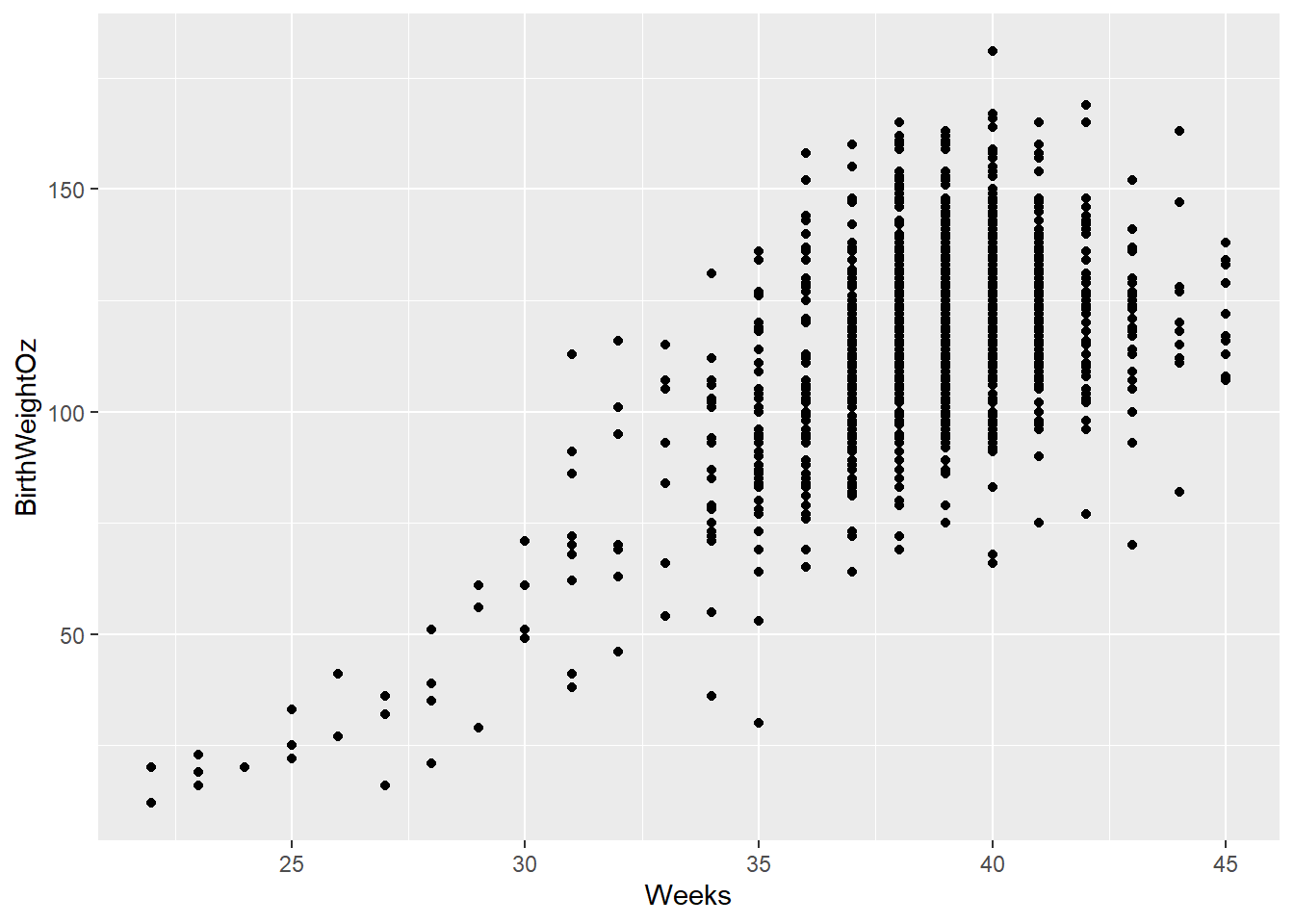``````# Boxplot of weight vs. weeks
ggplot(data = data,
aes(x = cut(Weeks, breaks = 5), y = BirthWeightOz)) +
geom_boxplot()``````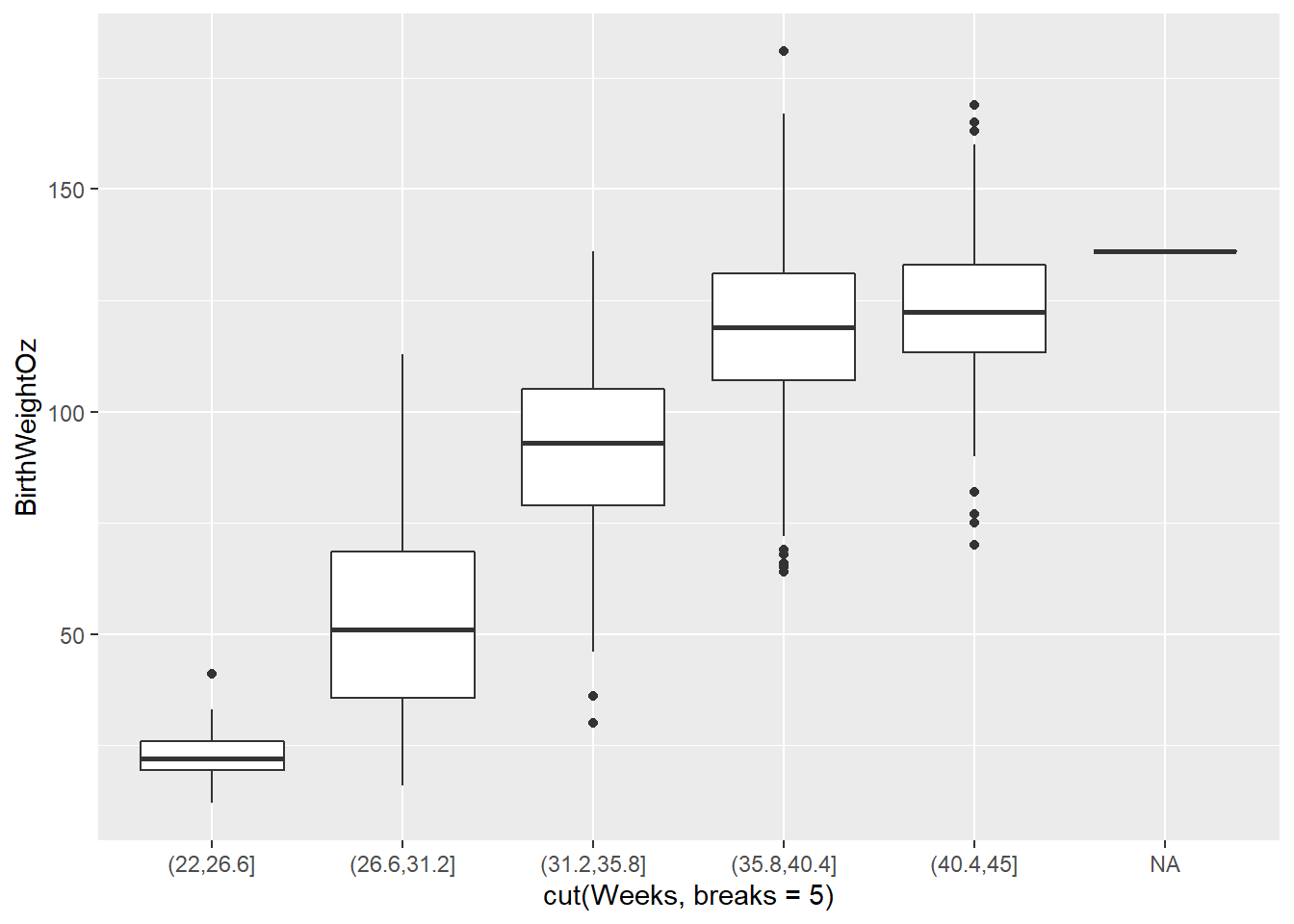``````
library(tidyverse)
## computing correlation

data %>%
summarize(N = n(), r = cor(BirthWeightOz, MomAge))
##      N         r
## 1 1450 0.1461145

# Compute correlation for all non-missing pairs
data %>%
summarize(N = n(), r = cor(BirthWeightOz, MomAge, use = "pairwise.complete.obs"))
##      N         r
## 1 1450 0.1461145``````
``````library(openintro)
##
## Attaching package: 'openintro'
## The following object is masked from 'package:ggplot2':
##
##     diamonds
## The following objects are masked from 'package:datasets':
##
##     cars, trees

ggplot(data = mammals, aes(y = BrainWt, x = BodyWt)) +
geom_point()``````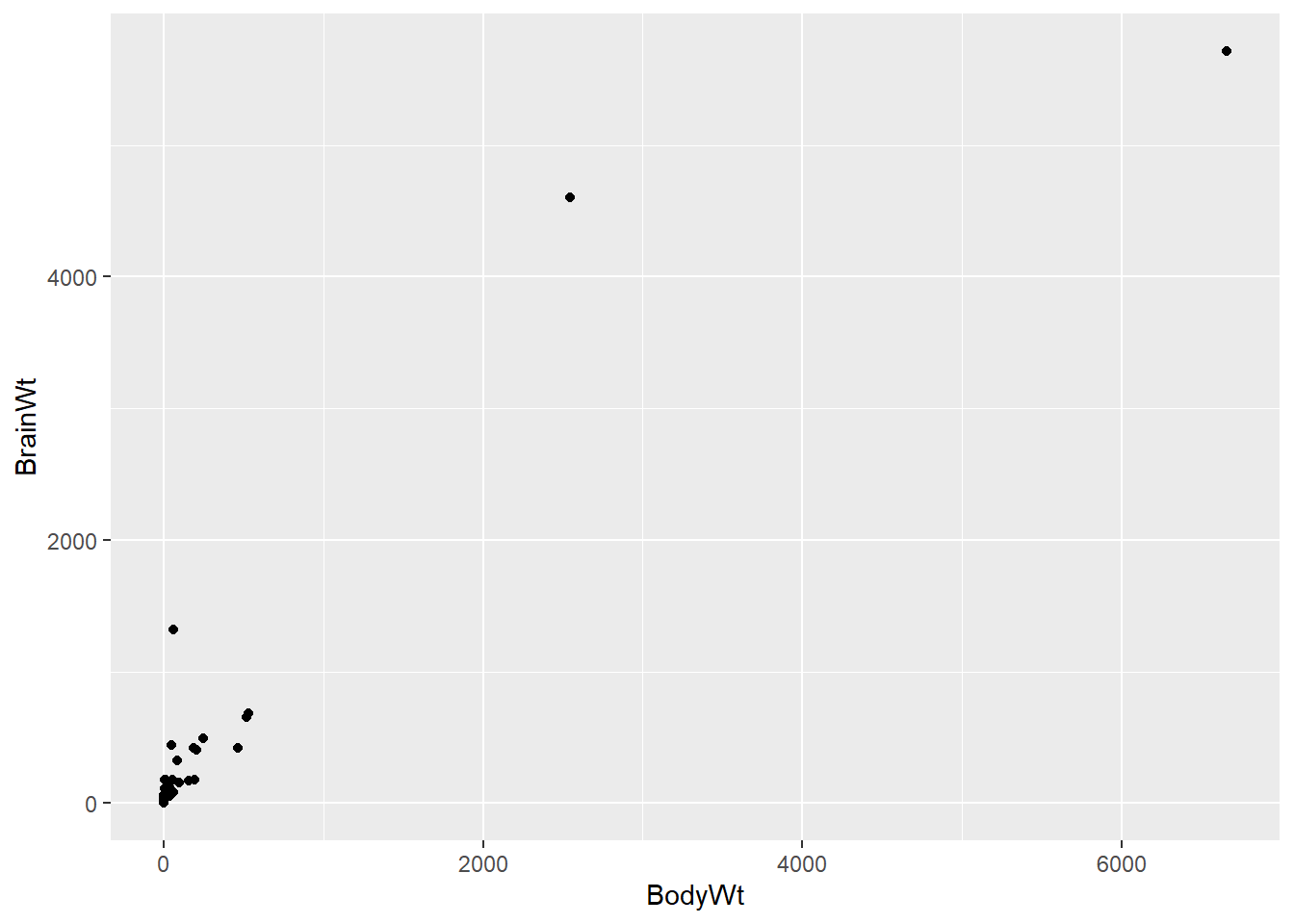``````
# Scatterplot with coord_trans()
ggplot(data = mammals, aes(y = BrainWt, x = BodyWt)) +
geom_point() +
coord_trans(x = "log10", y = "log10")``````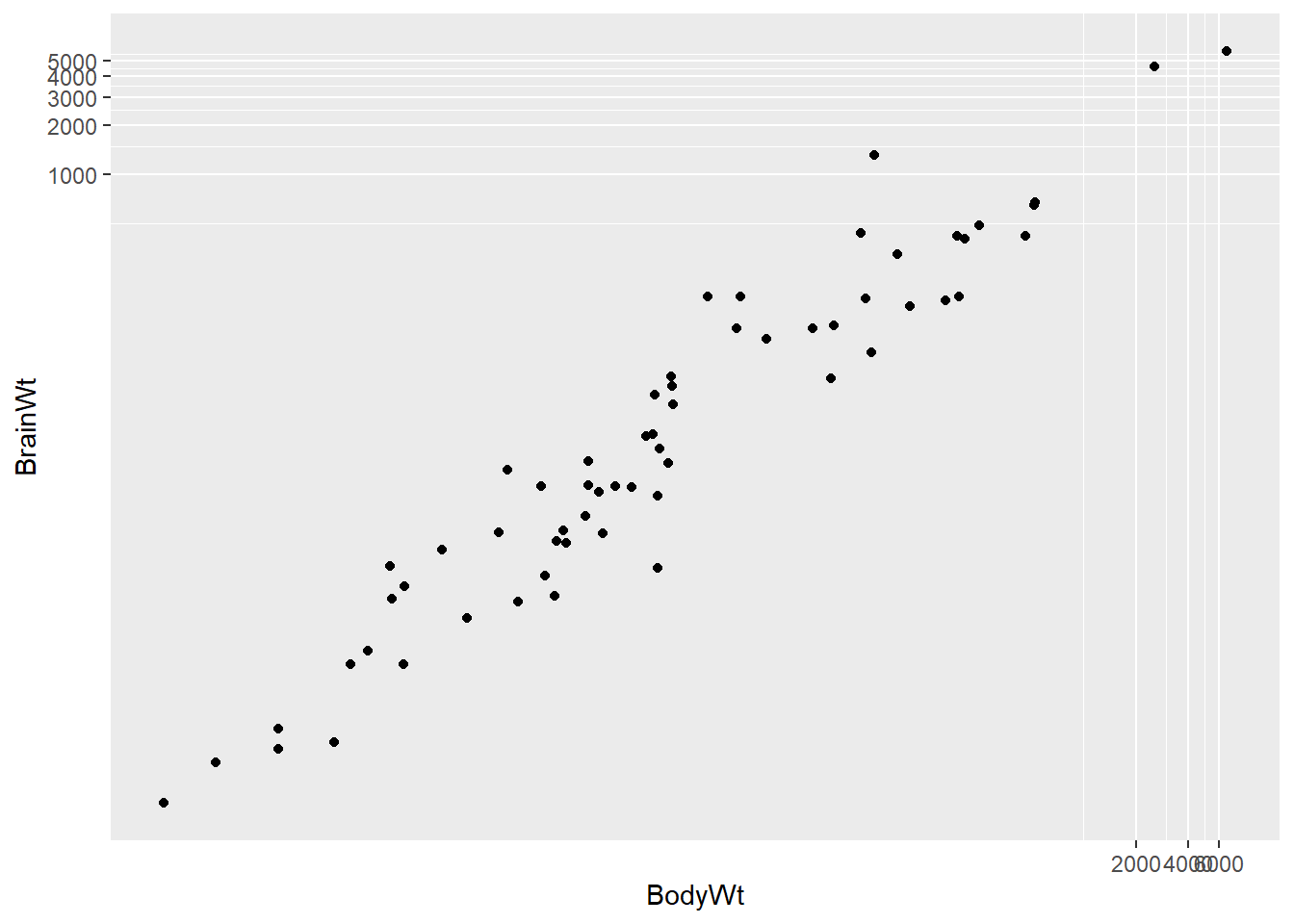``````
# Scatterplot with scale_x_log10() and scale_y_log10()
ggplot(data = mammals, aes(x = BodyWt, y = BrainWt)) +
geom_point() +
scale_x_log10() +
scale_y_log10()``````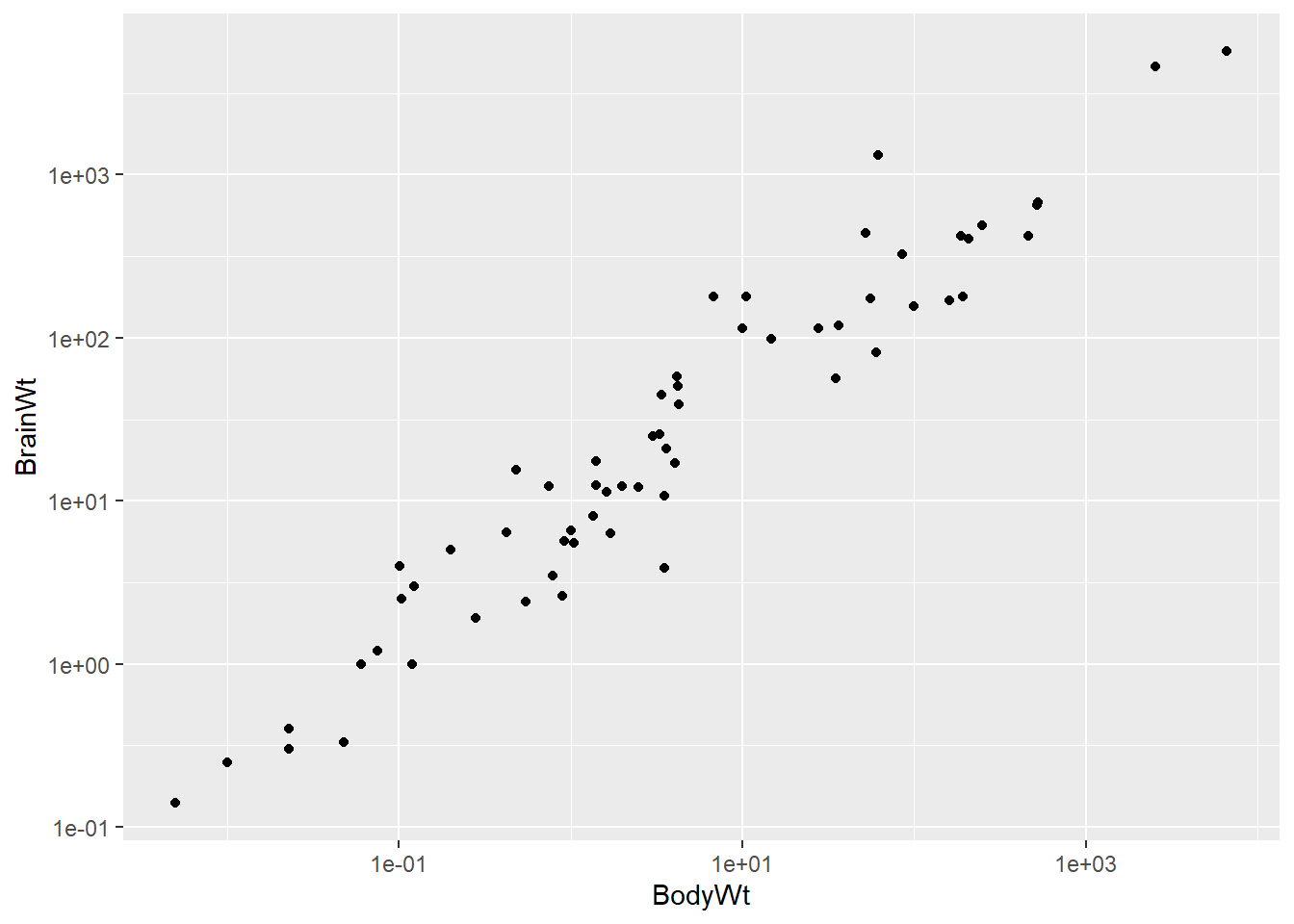``````
# Correlation among mammals, with and without log
mammals %>%
summarize(N = n(),
r = cor(BodyWt, BrainWt),
r_log = cor(log(BodyWt), log(BrainWt)))
##    N         r     r_log
## 1 62 0.9341638 0.9595748``````
``````library(tidyverse)
ggplot(data = mlbBat10, aes(y = SLG, x = OBP)) +
geom_point()``````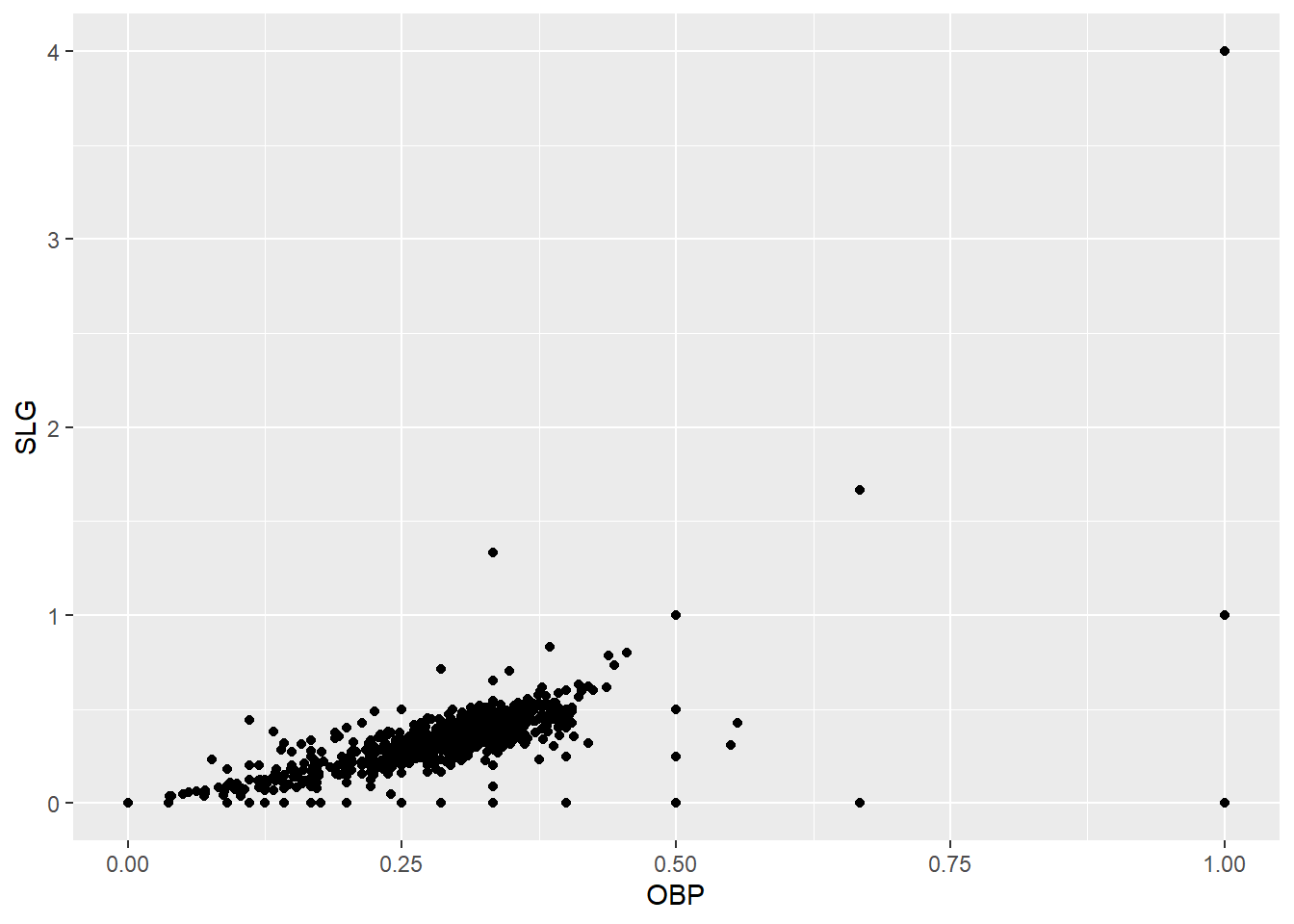``````
# identifying outliers
# Filter for AB greater than or equal to 200
ab_gt_200 <- mlbBat10 %>%
filter(AB >= 200)

# Scatterplot of SLG vs. OBP
ggplot(ab_gt_200, aes(x = OBP, y = SLG)) +
geom_point()``````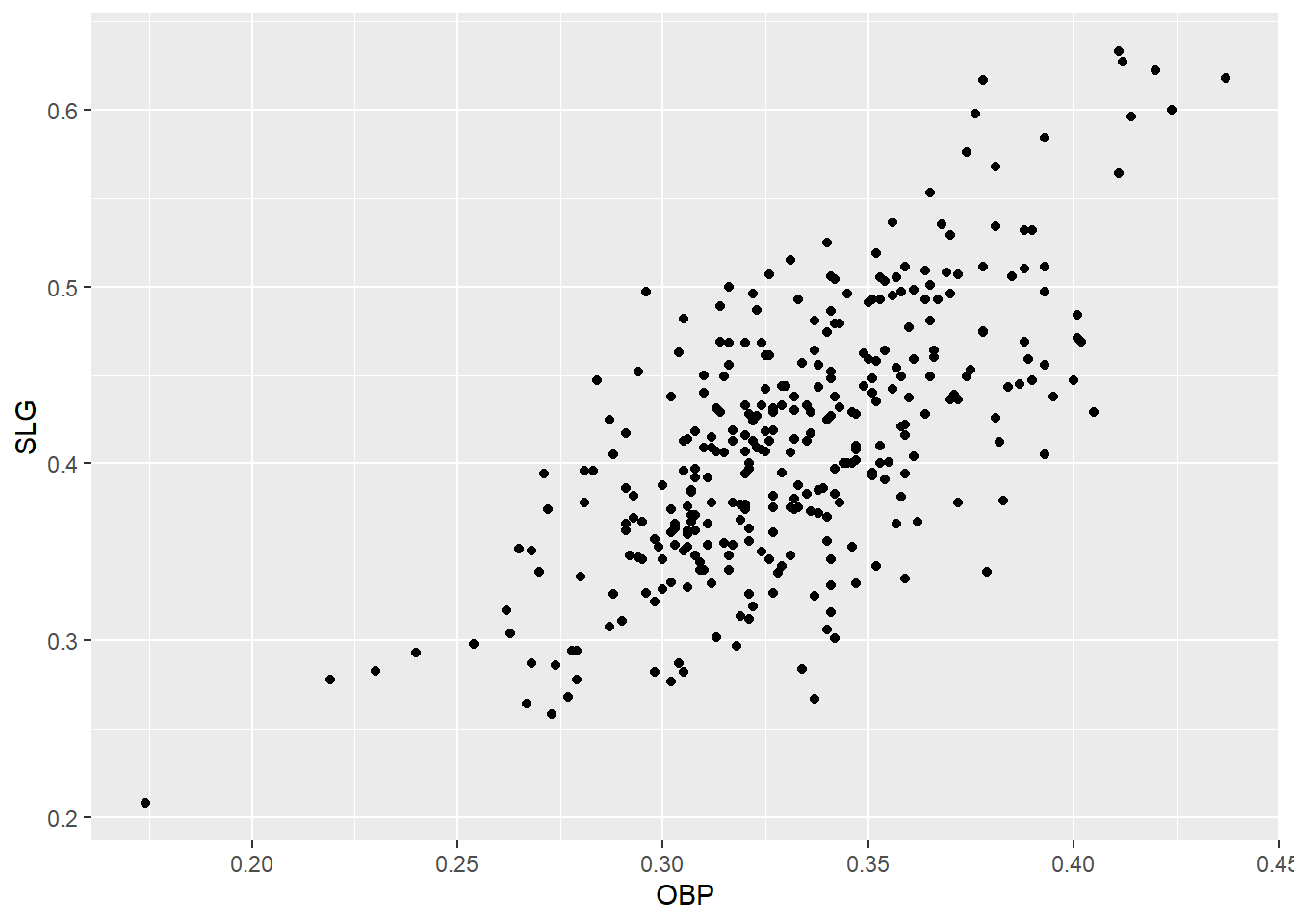``````
# Identify the outlying player
ab_gt_200 %>%
filter(OBP < 0.2)
##     name team position  G  AB  R  H 2B 3B HR RBI TB BB SO SB CS   OBP   SLG
## 1 B Wood  LAA       3B 81 226 20 33  2  0  4  14 47  6 71  1  0 0.174 0.208
##     AVG
## 1 0.146``````
``````

# Correlation for all baseball players
mlbBat10 %>%
summarize(N = n(), r = cor(OBP, SLG))
##      N         r
## 1 1199 0.8145628``````
``````
# Run this and look at the plot
mlbBat10 %>%
filter(AB > 200) %>%
ggplot(aes(x = OBP, y = SLG)) +
geom_point()``````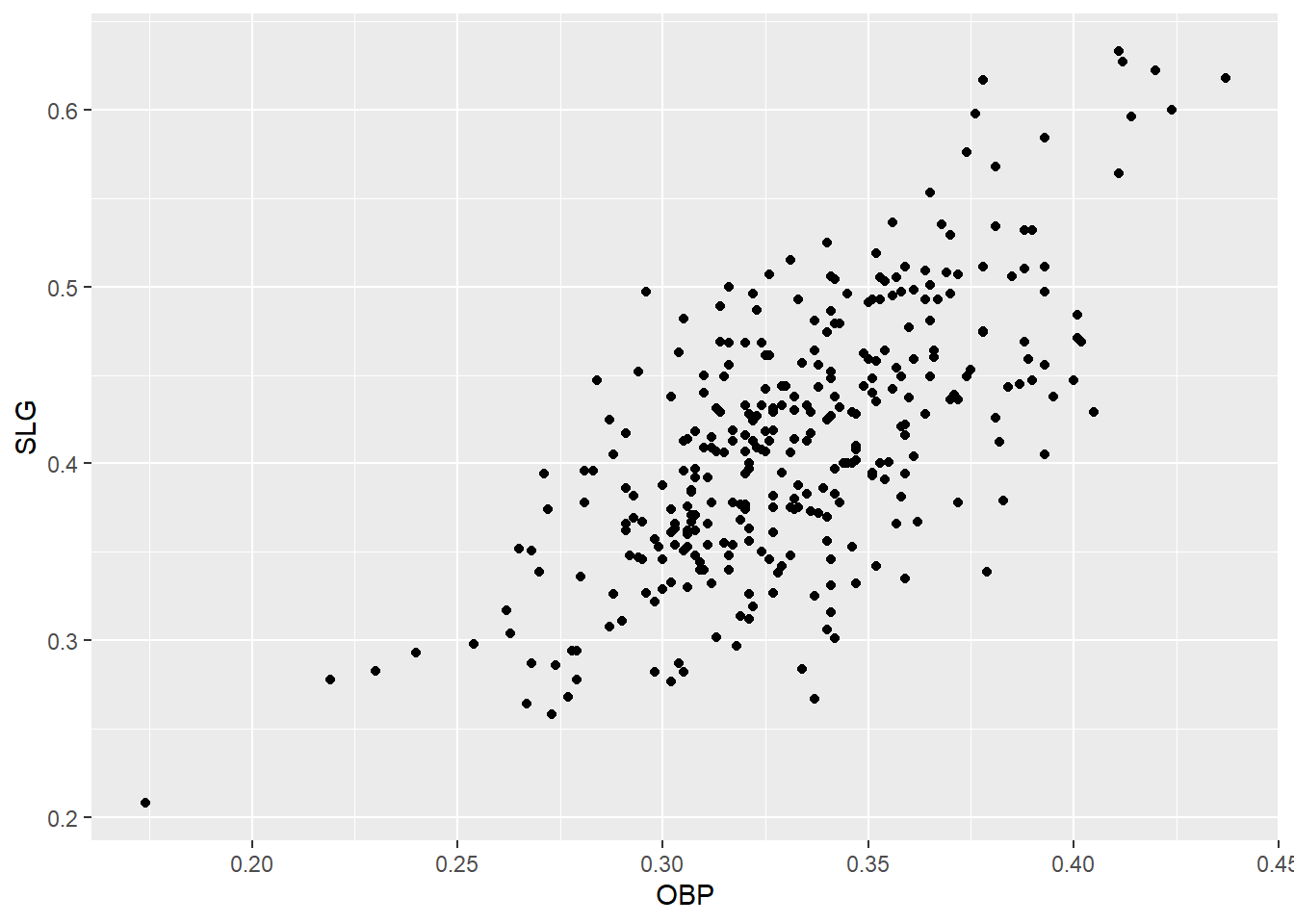``````
# Correlation for all players with at least 200 ABs
mlbBat10 %>%
filter(AB >= 200) %>%
summarize(N = n(), r = cor(OBP, SLG))
##     N         r
## 1 329 0.6855364``````
``````
# Run this and look at the plot
ggplot(data = bdims, aes(x = hgt, y = wgt, color = factor(sex))) +
geom_point() ````````````
# Correlation of body dimensions
bdims %>%
group_by(sex) %>%
summarize(N = n(), r = cor(hgt, wgt))
## # A tibble: 2 x 3
##     sex     N     r
##   <int> <int> <dbl>
## 1     0   260 0.431
## 2     1   247 0.535``````
``````
ggplot(data = smoking, aes(y = amtWeekdays, x = age)) +
geom_point()
## Warning: Removed 1270 rows containing missing values (geom_point).``````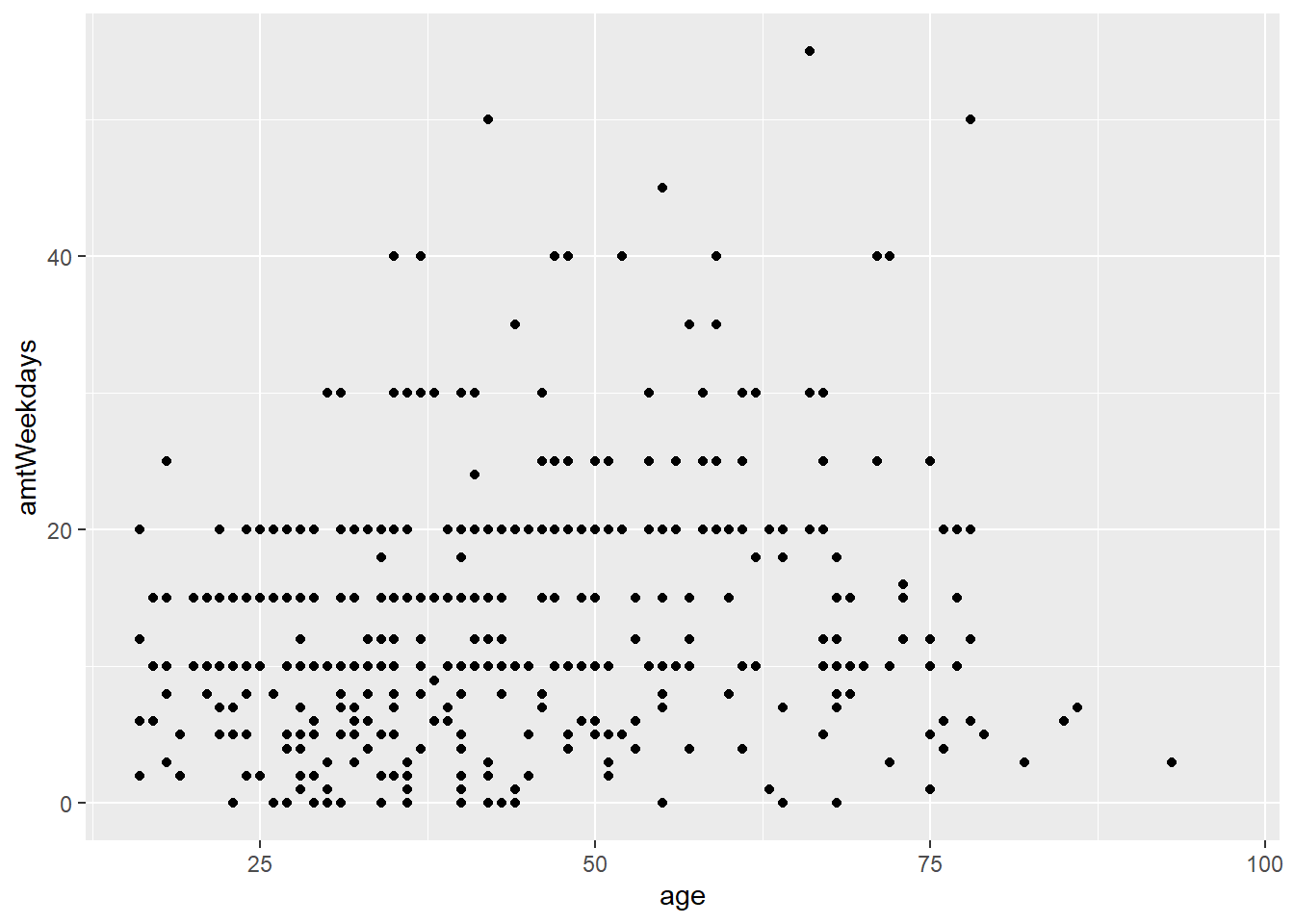``````
path1 <- "C:/Users/andre/OneDrive/Área de Trabalho/salerno/blogdown/datasets/anscombe"

path1 <- paste0(path1, "/anscombe.csv")

anscombe <- read.csv(path1, stringsAsFactors = FALSE, sep = ";")

# Compute properties of Anscombe
anscombe %>%
group_by(set) %>%
summarize(
N = n(),
mean_of_x = mean(x),
std_dev_of_x = sd(x),
mean_of_y = mean(y),
std_dev_of_y = sd(y),
correlation_between_x_and_y = cor(x, y)
)
## # A tibble: 4 x 7
##     set     N mean_of_x std_dev_of_x mean_of_y std_dev_of_y correlation_between…
##   <int> <int>     <dbl>        <dbl>     <dbl>        <dbl>                <dbl>
## 1     1    11         9         3.32      7.50         2.03                0.816
## 2     2    11         9         3.32      7.50         2.03                0.816
## 3     3    11         9         3.32      7.5          2.03                0.816
## 4     4    11         9         3.32      7.50         2.03                0.817``````